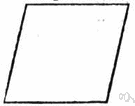# plane geometry

(redirected from Two-dimensional geometry)
Also found in: Thesaurus, Encyclopedia.

## plane geometry

n.
The geometry of planar figures.

## plane geometry

n
(Mathematics) the study of the properties of and relationships between plane curves, figures, etc

## plane′ geom′etry

n.
the geometry of figures whose parts all lie in one plane.
[1740–50]

## plane geometry

The mathematical study of geometric figures whose parts lie in the same plane, such as polygons, circles, and lines.
ThesaurusAntonymsRelated WordsSynonymsLegend:
 Noun 1plane geometry - the geometry of 2-dimensional figuresmath, mathematics, maths - a science (or group of related sciences) dealing with the logic of quantity and shape and arrangementgeometry - the pure mathematics of points and lines and curves and surfaces
Translations

## plane geometry

n (Math) → Planimetrie f
Site: Follow: Share:
Open / Close